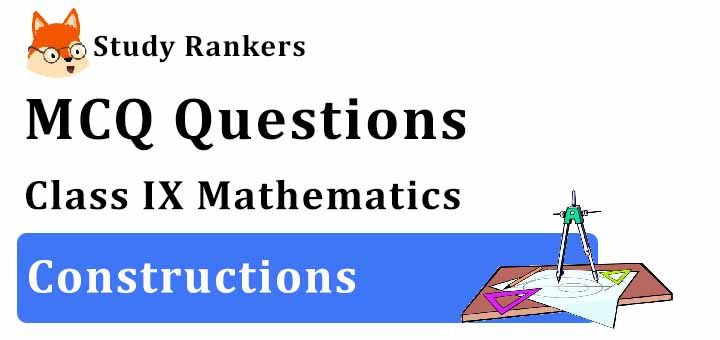## MCQ Questions for Class 9 Maths: Ch 11 Constructions1. Which of the following angles can be constructed using ruler and compasses?
(a) 35°
(b) 45°
(c) 95°
(d) 55°
► (b) 45°

2. With the help of a rular and compass, it is not possible to construct an angle of
(a) 35°
(b) 67.5°
(c) 82.5°
(d) 7.5°
► (a) 35°

3. With the help of a ruler and compass, it is possible to construct an angle of
(a) 40°
(b) 37.5°
(c) 65°
(d) 50°
► (b) 37.5°

4. To construct a ΔABC in which BC = 10 cm and ∠B= 60 degrees and AB + AC = 14 cm, then the length of BD used for construction.
(a) 7 cm
(b) 14 cm
(c) 20 cm
(d) 10 cm
►(b) 14 cm

5. An angle whose measure is more than 180° and less than 360° is called a
(a) Reflex angle
(b) Acute angle
(c) Straight angle
(d) Complete angle
► (a) Reflex angle

6. If two circles touches internally then distance between their centres is equal to
(b) not possible to determine
(d) none

7. On a ray AB with initial point A, Taking A as centre and some radius, draw an arc of a circle, which intersects AB, say at a point D. Taking D as centre and with the same radius as before, draw an arc intersecting the previously drawn arc, say at a point E. Draw the ray AC passing through E. Then, the measure of ∠CAB is
(a) 30°
(b) 60°
(c) 45°
(d) 15°
► (b) 60°

8. The bisector of an angle lies in its
(a) Interior
(b) On the arms of the angle
(c) Any where in the plane
(d) Exterior
► (a) Interior

9. If two circles touches internally then distance between their centres is equal to
(c) not possible to determine
(d) none

10. The internal and external bisectors of an angle form a
(a) Acute angle
(b) Straight angle
(c) Right angle
(d) Reflex angle
► (c) Right angle

11. Two radii of same circle are always :
(a) may inchired at any angle
(b) perpendicular
(c) parallel
(d) parallel and may inchired at any angle
► (d) parallel and may inchired at any angle

12. Which of these angles cannot be constructed using ruler and compasses?
(a) 120°
(b) 60°
(c) 140°
(d) 135°
► (c. 140°

13. Which of the following angles can be constructed using ruler and compass?
(a) 35°
(b) 40°
(c) 90°
(d) 50°
► (c) 90°

14. On a ray AB with initial point A, Taking A as centre and some radius, draw an arc of a circle, which intersects AB, say at a point D. Taking D as centre and with the same radius as before, draw an arc intersecting the previously drawn arc, say at a point E. Draw the ray AC passing through E. Then, the measure of ∠CAB is
(a) 15°
(b) 30°
(c) 45°
(d) 60°
► (d) 60°

15. The construction of △ABC, given that BC = 5 cm, ∠B = 600 is not possible when the difference of AB and AC is equal to
(a) 4.2 cm
(b) 5.9 cm.
(c) 4 cm.
(d) 3 cm.
► (b) 5.9 cm.

16. The construction of a triangle ABC with AB = 4 cm and ∠A = 60° is not possible when difference of BC and AC is equal to
(a) 3.5 cm
(b) 4.5 cm
(c) 2.5 cm
(d) 3 cm
► (b) 4.5 cm

17. With the help of a ruler and a compass, it is possible to construct an angle of
(a) 40°
(b) 37.5°
(c) 47.5°
(d) 35°
► (b) 37.5°

18. The point of concurrence of the three angle bisectors of a triangle, is called
(a) Centroid
(b) Incentre
(c) Circumcentre
(d) Orthocentre
► (b) Incentre

19. In Δ ABC, which of the following information is needed to construct it if it is known that measure of ∠B = 60 and BC = 6 cm :
(a) AB + BC
(b) CA + AB
(c) BC + CA
(d) All of the above
► (d) All of the above

20. An external bisector of an angle measuring 70° will divide the angle into two angles measuring
(a) 35°
(b) 55°
(c) 70°
(d) 110°
► (b) 55°

21. Two radii of the same circle are always:
(a) may inchired at any angle
(b) parallel
(c) parallel and may inchired at any angle
(d) perpendicular
► (c) parallel and may inchired at any angle

22. In Δ ABC if ∠B = ∠C = 300, which of the following is the longest side?
(a) BC
(b) AC
(c) AB
(d) none
► (a) BC